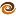# EmptyCrate

#### About Me | Contact Me | Training |ChaiScript.com | YouTube Channel

On occasion you will read or hear someone talking about C++ templates causing code bloat. I was thinking about it the other day and thought to myself, “self, if the code does exactly the same thing then the compiled code cannot really be any bigger, can it?” Here are the test cases presented, first without the use of templates, second with the use of templates. Exactly the same functionality and exactly the same code output:

``````#include <iostream>

void print(int i)
{
std::cout << i << std::endl;
}

void print(const std::string &s)
{
std::cout << s << std::endl;
}

void print(double d)
{
std::cout << d << std::endl;
}

void print(bool b)
{
std::cout << b << std::endl;
}

int main()
{
print(1);
//Note, I have to put it in a std::string() otherwise the compiler thinks it's a const char *
//which gets converted to an int or bool or something
print(std::string("hello world"));
print(4.5);
print(false);
}
``````

And with the use of templates:

``````#include <iostream>

template
void print(const T &t)
{
std::cout << t << std::endl;
}

int main()
{
print(1);
print(std::string("hello world"));
print(4.5);
print(false);
}
``````

There is no question that the templated version is smaller, easier to maintain and easier to grok than the first version (assuming a basic understanding of templates). They both produce exactly the same output:

``````1
hello world
4.5
0
``````

And what about compiled code size? Each were compiled with the command `g++ .cpp -O3`.

Non-template version: 8140 bytes, template version: 8028 bytes!

The compiled size of the templated version was smaller. In the interest of full disclosure, with anything compiled less than `-O3`, the template version is 20 bytes larger than the non-template version. Also, build times do not vary between the two versions. Each takes approximately .623 seconds to compile.

So, what are we seeing here, really? Templates do not “cause code bloat” or long compile times. The fact is, if one were to write the same exact code with both templates and non-template versions the compile times and resulting code size would probably be very close. However, the program sources for the non-template versions would be so insurmountably large they would likely be unmaintainable.

For example, if one were to use a `std::vector<std::string>`, `std::vector<float>` and `std::vector< std::vector<float> >` in his code, the resulting compiled code would be large indeed, as the compiler would generate no less than 4 versions of vector for him. However, if he were to hand write `string_vector`, `int_vector`, `float_vector` and `float_vector_vector` the amount of code to maintain would be huge. Bugs found in `string_vector` would more than likely not get fixed in `int_vector`, and the compiled code would almost assuredly be the same size as the standard template versions.

My Videos

Related